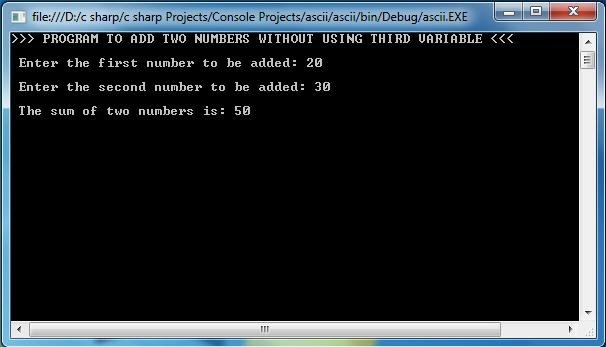# C# PROGRAM TO ADD TWO INTEGERS USING METHOD

### C# PROGRAM TO ADD TWO INTEGERS USING METHOD :

```namespace CsharpPrograms
{
class Program
{
static void Main(string[] args)
{

int x,y,result=0;
Console.WriteLine(">>> PROGRAM TO ADD TWO NUMBERS WITHOUT USING THIRD VARIABLE <<< ");
Console.Write("\n Enter the first number to be added: ");
x=Convert.ToInt32(Console.ReadLine());         // taking x value from keyboard
Console.Write("\n Enter the second number to be added: ");
y = Convert.ToInt32(Console.ReadLine());         // taking y value from keyboard

Console.WriteLine("\n The sum of two numbers is: {0} ",result);    /*printing the sum.*/
}

static public int Add(int a, int b)
{
int result = a + b;
return result;
}
}

}```

Sample Output:Explanation :
In the above program the function static public int Add(int a,int b) , we are declaring function as Static . Because, c# is a Object Oriented Programming Language. And in OOP language, a function can be called using object of a class. And if we want to call function without creating object, we have to declare it as static.

For C Program : C Program to Add Two Numbers Using Function